Publicité

# Risk & capital budgeting

19 Mar 2018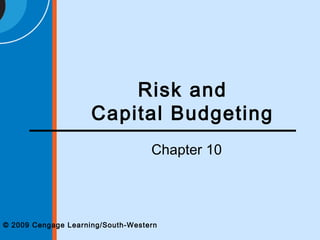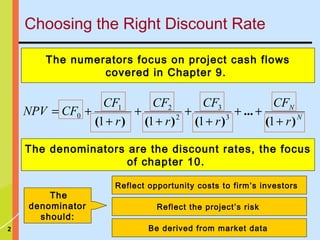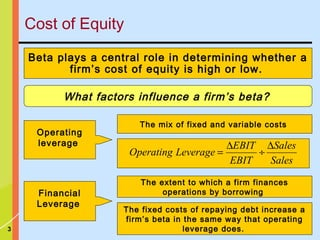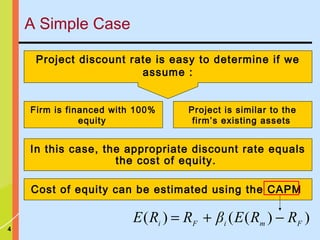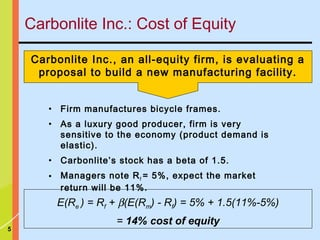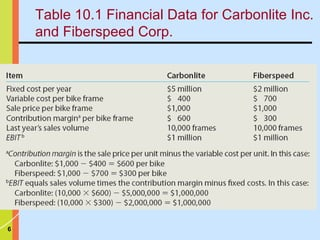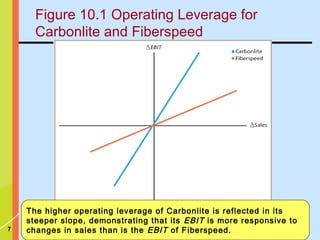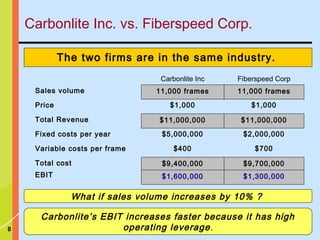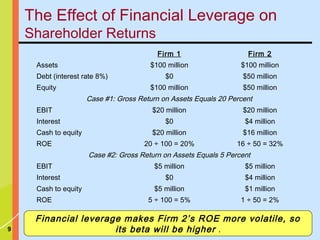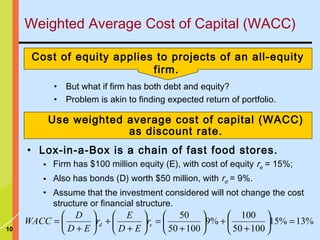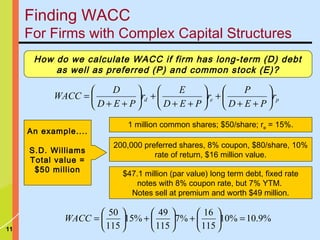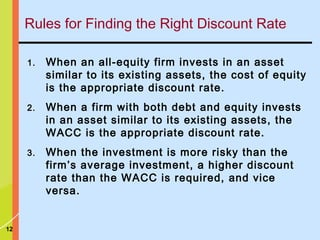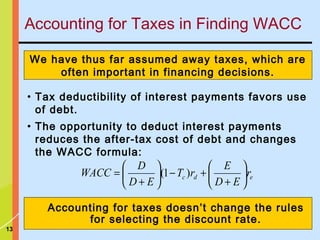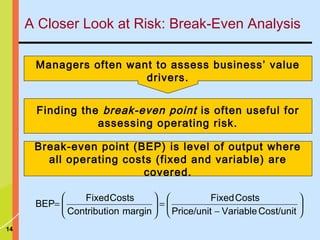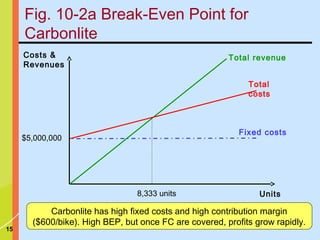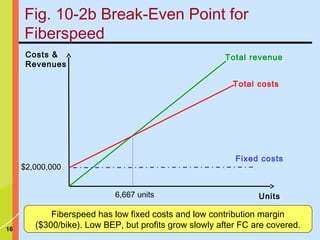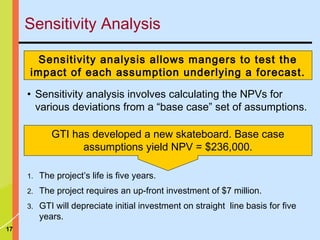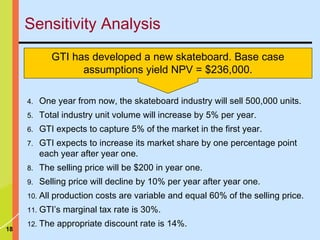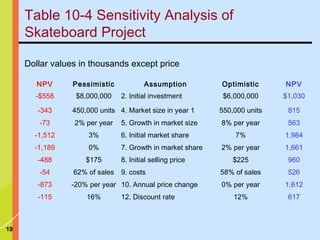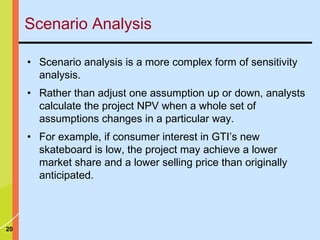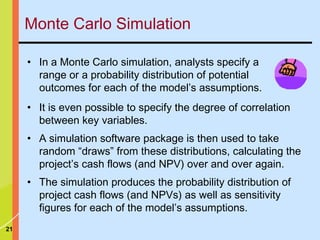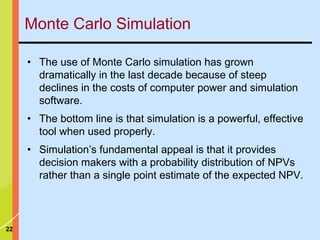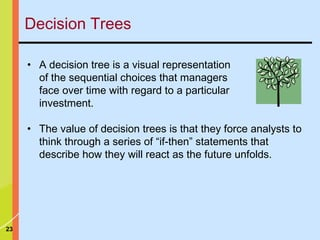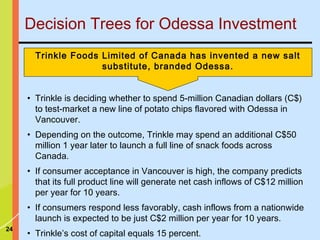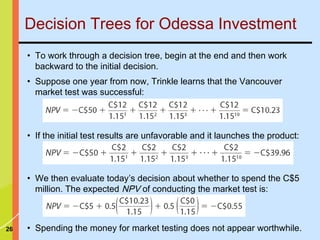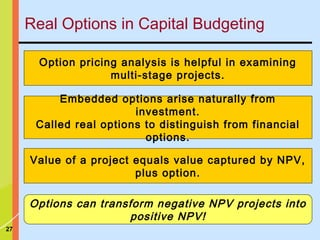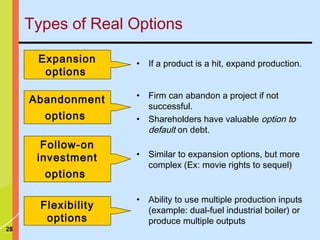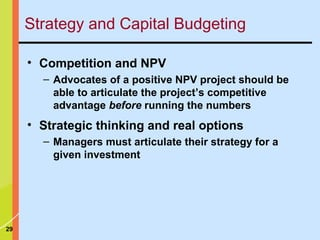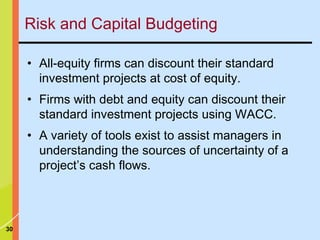1 sur 30
Publicité

### Risk & capital budgeting

1. © 2009 Cengage Learning/South-Western Risk and Capital Budgeting Chapter 10
2. 2 N N r CF r CF r CF r CF CFNPV )( ... )()()( + ++ + + + + + += 1111 3 3 2 21 0 The numerators focus on project cash flows covered in Chapter 9. The denominators are the discount rates, the focus of chapter 10. The denominator should: Reflect opportunity costs to firm’s investors Reflect the project’s risk Be derived from market data Choosing the Right Discount Rate
3. 3 Beta plays a central role in determining whether a firm’s cost of equity is high or low. What factors influence a firm’s beta? Operating leverage The mix of fixed and variable costs Sales Sales EBIT EBIT LeverageOperating ∆ ÷ ∆ = Financial Leverage The extent to which a firm finances operations by borrowing The fixed costs of repaying debt increase a firm’s beta in the same way that operating leverage does. Cost of Equity
4. 4 Firm is financed with 100% equity Project is similar to the firm’s existing assets Project discount rate is easy to determine if we assume : In this case, the appropriate discount rate equals the cost of equity. Cost of equity can be estimated using the CAPM ))(()( FmiFi RREβRRE −+= A Simple Case
5. 5 E(Re ) = Rf + β(E(Rm) - Rf) = 5% + 1.5(11%-5%) = 14% cost of equity Carbonlite Inc., an all-equity firm, is evaluating a proposal to build a new manufacturing facility. • Firm manufactures bicycle frames. • As a luxury good producer, firm is very sensitive to the economy (product demand is elastic). • Carbonlite’s stock has a beta of 1.5. • Managers note Rf = 5%, expect the market return will be 11%. Carbonlite Inc.: Cost of Equity
6. 6 Table 10.1 Financial Data for Carbonlite Inc. and Fiberspeed Corp.
7. 7 Figure 10.1 Operating Leverage for Carbonlite and Fiberspeed The higher operating leverage of Carbonlite is reflected in its steeper slope, demonstrating that its EBIT is more responsive to changes in sales than is the EBIT of Fiberspeed.
8. 8 Carbonlite Inc Fiberspeed Corp Sales volume 10,000 sofas 10,000 sofas Price \$1,000 \$1,000 Total Revenue \$10,000,000 \$10,000,000 Fixed costs per year \$5,000,000 \$2,000,000 Variable costs per frame \$400 \$700 Total cost \$9,000,000 \$9,000,000 EBIT \$1,000,000 \$1,000,000 What if sales volume increases by 10% ? 11,000 frames11,000 frames \$11,000,000\$11,000,000 \$9,700,000\$9,400,000 \$1,300,000\$1,600,000 The two firms are in the same industry. Carbonlite’s EBIT increases faster because it has high operating leverage. Carbonlite Inc. vs. Fiberspeed Corp.
9. 9 Firm 1 Firm 2 Assets \$100 million \$100 million Debt (interest rate 8%) \$0 \$50 million Equity \$100 million \$50 million Case #1: Gross Return on Assets Equals 20 Percent EBIT \$20 million \$20 million Interest \$0 \$4 million Cash to equity \$20 million \$16 million ROE 20 ÷ 100 = 20% 16 ÷ 50 = 32% Case #2: Gross Return on Assets Equals 5 Percent EBIT \$5 million \$5 million Interest \$0 \$4 million Cash to equity \$5 million \$1 million ROE 5 ÷ 100 = 5% 1 ÷ 50 = 2% Financial leverage makes Firm 2’s ROE more volatile, so its beta will be higher . The Effect of Financial Leverage on Shareholder Returns
10. 10 Cost of equity applies to projects of an all-equity firm. • But what if firm has both debt and equity? • Problem is akin to finding expected return of portfolio. Use weighted average cost of capital (WACC) as discount rate. %13%15 10050 100 %9 10050 50 =      + +      + =      + +      + = ed r ED E r ED D WACC • Lox-in-a-Box is a chain of fast food stores. • Firm has \$100 million equity (E), with cost of equity re = 15%; • Also has bonds (D) worth \$50 million, with rd = 9%. • Assume that the investment considered will not change the cost structure or financial structure. Weighted Average Cost of Capital (WACC)
11. 11 ped r PED P r PED E r PED D WACC       ++ +      ++ +      ++ = %9.10%10 115 16 %7 115 49 %15 115 50 =      +      +      =WACC How do we calculate WACC if firm has long-term (D) debt as well as preferred (P) and common stock (E)? An example.... S.D. Williams Total value = \$50 million 1 million common shares; \$50/share; re = 15%. 200,000 preferred shares, 8% coupon, \$80/share, 10% rate of return, \$16 million value. \$47.1 million (par value) long term debt, fixed rate notes with 8% coupon rate, but 7% YTM. Notes sell at premium and worth \$49 million. Finding WACC For Firms with Complex Capital Structures
12. 12 Rules for Finding the Right Discount Rate 1. When an all-equity firm invests in an asset similar to its existing assets, the cost of equity is the appropriate discount rate. 2. When a firm with both debt and equity invests in an asset similar to its existing assets, the WACC is the appropriate discount rate. 3. When the investment is more risky than the firm’s average investment, a higher discount rate than the WACC is required, and vice versa.
13. 13 edc r ED E rT ED D WACC       + +−      + = )1( We have thus far assumed away taxes, which are often important in financing decisions. • Tax deductibility of interest payments favors use of debt. • The opportunity to deduct interest payments reduces the after-tax cost of debt and changes the WACC formula: Accounting for taxes doesn’t change the rules for selecting the discount rate. Accounting for Taxes in Finding WACC
14. 14 Managers often want to assess business’ value drivers. Finding the break-even point is often useful for assessing operating risk. Break-even point (BEP) is level of output where all operating costs (fixed and variable) are covered.       − =      = Cost/unitVariablePrice/unit CostsFixed marginonContributi CostsFixed BEP A Closer Look at Risk: Break-Even Analysis
15. 15 \$5,000,000 Total revenue Total costs Fixed costs Units8,333 units Costs & Revenues Carbonlite has high fixed costs and high contribution margin (\$600/bike). High BEP, but once FC are covered, profits grow rapidly. Fig. 10-2a Break-Even Point for Carbonlite
16. 16 \$2,000,000 Total revenue Total costs Fixed costs Units6,667 units Costs & Revenues Fiberspeed has low fixed costs and low contribution margin (\$300/bike). Low BEP, but profits grow slowly after FC are covered. Fig. 10-2b Break-Even Point for Fiberspeed
17. 17 Sensitivity analysis allows mangers to test the impact of each assumption underlying a forecast. • Sensitivity analysis involves calculating the NPVs for various deviations from a “base case” set of assumptions. GTI has developed a new skateboard. Base case assumptions yield NPV = \$236,000. 1. The project’s life is five years. 2. The project requires an up-front investment of \$7 million. 3. GTI will depreciate initial investment on straight line basis for five years. Sensitivity Analysis
18. 18 4. One year from now, the skateboard industry will sell 500,000 units. 5. Total industry unit volume will increase by 5% per year. 6. GTI expects to capture 5% of the market in the first year. 7. GTI expects to increase its market share by one percentage point each year after year one. 8. The selling price will be \$200 in year one. 9. Selling price will decline by 10% per year after year one. 10. All production costs are variable and equal 60% of the selling price. 11. GTI’s marginal tax rate is 30%. 12. The appropriate discount rate is 14%. Sensitivity Analysis GTI has developed a new skateboard. Base case assumptions yield NPV = \$236,000.
19. 19 NPV Pessimistic Assumption Optimistic NPV -\$558 \$8,000,000 2. Initial investment \$6,000,000 \$1,030 -343 450,000 units 4. Market size in year 1 550,000 units 815 -73 2% per year 5. Growth in market size 8% per year 563 -1,512 3% 6. Initial market share 7% 1,984 -1,189 0% 7. Growth in market share 2% per year 1,661 -488 \$175 8. Initial selling price \$225 960 -54 62% of sales 9. costs 58% of sales 526 -873 -20% per year 10. Annual price change 0% per year 1,612 -115 16% 12. Discount rate 12% 617 Dollar values in thousands except price Table 10-4 Sensitivity Analysis of Skateboard Project
20. 20 Scenario Analysis • Scenario analysis is a more complex form of sensitivity analysis. • Rather than adjust one assumption up or down, analysts calculate the project NPV when a whole set of assumptions changes in a particular way. • For example, if consumer interest in GTI’s new skateboard is low, the project may achieve a lower market share and a lower selling price than originally anticipated.
21. 21 Monte Carlo Simulation • In a Monte Carlo simulation, analysts specify a range or a probability distribution of potential outcomes for each of the model’s assumptions. • It is even possible to specify the degree of correlation between key variables. • A simulation software package is then used to take random “draws” from these distributions, calculating the project’s cash flows (and NPV) over and over again. • The simulation produces the probability distribution of project cash flows (and NPVs) as well as sensitivity figures for each of the model’s assumptions.
22. 22 Monte Carlo Simulation • The use of Monte Carlo simulation has grown dramatically in the last decade because of steep declines in the costs of computer power and simulation software. • The bottom line is that simulation is a powerful, effective tool when used properly. • Simulation’s fundamental appeal is that it provides decision makers with a probability distribution of NPVs rather than a single point estimate of the expected NPV.
23. 23 Decision Trees • A decision tree is a visual representation of the sequential choices that managers face over time with regard to a particular investment. • The value of decision trees is that they force analysts to think through a series of “if-then” statements that describe how they will react as the future unfolds.
24. 24 • Trinkle is deciding whether to spend 5-million Canadian dollars (C\$) to test-market a new line of potato chips flavored with Odessa in Vancouver. • Depending on the outcome, Trinkle may spend an additional C\$50 million 1 year later to launch a full line of snack foods across Canada. • If consumer acceptance in Vancouver is high, the company predicts that its full product line will generate net cash inflows of C\$12 million per year for 10 years. • If consumers respond less favorably, cash inflows from a nationwide launch is expected to be just C\$2 million per year for 10 years. • Trinkle’s cost of capital equals 15 percent. Decision Trees for Odessa Investment Trinkle Foods Limited of Canada has invented a new salt substitute, branded Odessa.
25. 25 If the test market is successful, the NPV of launching the product is C\$10.23 million; if the initial test results are negative, and it launches the product, it will have an NPV of – C\$39.96 million. Fig. 10-3 Decision Trees for Odessa Investment
26. 26 • To work through a decision tree, begin at the end and then work backward to the initial decision. • Suppose one year from now, Trinkle learns that the Vancouver market test was successful: • If the initial test results are unfavorable and it launches the product: • We then evaluate today’s decision about whether to spend the C\$5 million. The expected NPV of conducting the market test is: • Spending the money for market testing does not appear worthwhile. Decision Trees for Odessa Investment
27. 27 Embedded options arise naturally from investment. Called real options to distinguish from financial options. Option pricing analysis is helpful in examining multi-stage projects. Options can transform negative NPV projects into positive NPV! Value of a project equals value captured by NPV, plus option. Real Options in Capital Budgeting
28. 28 Expansion options • If a product is a hit, expand production. Abandonment options • Firm can abandon a project if not successful. • Shareholders have valuable option to default on debt. Follow-on investment options • Similar to expansion options, but more complex (Ex: movie rights to sequel) Flexibility options • Ability to use multiple production inputs (example: dual-fuel industrial boiler) or produce multiple outputs Types of Real Options
29. 29 Strategy and Capital Budgeting • Competition and NPV – Advocates of a positive NPV project should be able to articulate the project’s competitive advantage before running the numbers • Strategic thinking and real options – Managers must articulate their strategy for a given investment
30. 30 • All-equity firms can discount their standard investment projects at cost of equity. • Firms with debt and equity can discount their standard investment projects using WACC. • A variety of tools exist to assist managers in understanding the sources of uncertainty of a project’s cash flows. Risk and Capital Budgeting
Publicité